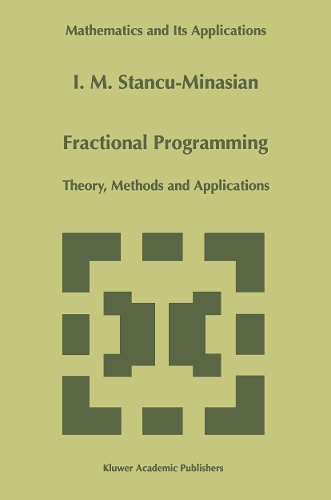•# Fractional Programming: Theory, Methods and Applications - Mathematics and Its Applications 409 (Paperback)

(author)
£79.99
Paperback 432 Pages / Published: 17/09/2011
• We can order this

Usually dispatched within 3 weeks

Mathematical programming has know a spectacular diversification in the last few decades. This process has happened both at the level of mathematical research and at the level of the applications generated by the solution methods that were created. To write a monograph dedicated to a certain domain of mathematical programming is, under such circumstances,especially difficult. In the present monograph we opt for the domain of fractional programming. Interest of this subject was generated by the fact that various optimization problems from engineering and economics consider the minimization of a ratio between physical and/or economical functions, for example cost/time, cost/volume,cost/profit, or other quantities that measure the efficiency of a system. For example, the productivity of industrial systems, defined as the ratio between the realized services in a system within a given period of time and the utilized resources, is used as one of the best indicators of the quality of their operation. Such problems, where the objective function appears as a ratio of functions, constitute fractional programming problem. Due to its importance in modeling various decision processes in management science, operational research, and economics, and also due to its frequent appearance in other problems that are not necessarily economical, such as information theory, numerical analysis, stochastic programming, decomposition algorithms for large linear systems, etc., the fractional programming method has received particular attention in the last three decades.

Publisher: Springer
ISBN: 9789401065047
Number of pages: 432
Weight: 686 g
Dimensions: 240 x 160 x 30 mm
Edition: Softcover reprint of the original 1st ed. 199